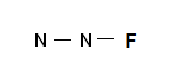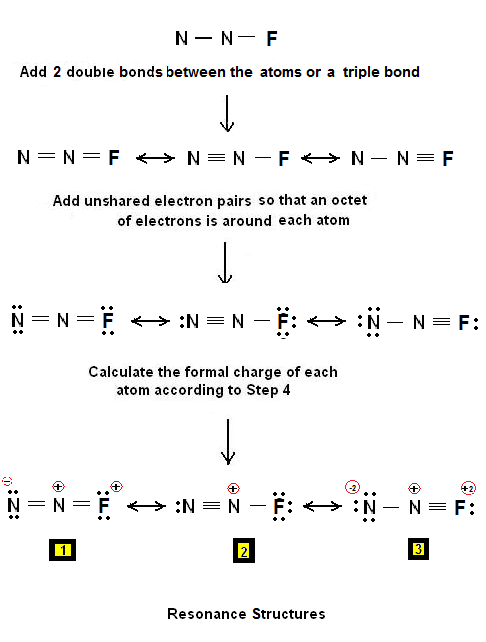A Brief Tutorial on drawing Lewis Structures of the N2F+ | Chemistry Net

# A Brief Tutorial on drawing Lewis Structures of the N2F+

A Brief Tutorial on drawing electron dot structures of the N2F+

# A Brief Tutorial on drawing Electron Dot Structures of the N2F+ cation

A simple procedure for writing Lewis structures is given in a previous article entitled “Lewis Structures and the Octet Rule”. Several worked examples relevant to this procedure were given in previous posts please see the Sitemap - Table of Contents (Lewis Electron Dot Structures).

Another example  for writing Lewis structures following the above procedure is given below.

Let us consider the case of the electron dot structures of N2F+cation. The N2F+ cation is of great interest. Force field studies and ab initio calculations suggested that this cation should possess an an unusually short N-F bond. The results of STO-6G valence bond (VB) calculations for the linear cation N2F+ show that (a) the dominant Lewis-type VB structures involve a single positive charge which is located on either of the nitrogen atoms, and (b) a small amount of N–F π-bonding arises primarily from the formation of one-electron N–F π-bonds. It is suggested that N–F π-bonding is one of several factors which are responsible for the shortening of the N–F bond of N2F+ .

Let us draw the N2F+ electron dot structures?

Step 1: Connect the atoms with single bonds:Step 2: Calculate the # of electrons in π bonds (multiple bonds) using  formula (1) in the article entitled “Lewis Structures and the Octet Rule”.

Where n in this case is 3 since N2F+ consists of 3 atoms. Where V = (2*5 + 7 ) - charge = 17 – 1 = 16

Therefore, P = 6n + 2 – V = 6 *3 + 2 – 16 = 4

Therefore, there are: 1) either 2 double bonds 2) or 1 triple bond

Step 3 & 4: The Lewis structures for N2F+ are as follows:Therefore, three plausible electron dot structures were drawn for the N2F+ cation. The least plausible structures are #1 (adjacent + charges ) and #3 (large charge separation)

Relevant Posts - Relevant Videos

Lewis Structures|Octet Rule: A Simple Method to write Lewis Structures

Simple Method for writing Lewis Structures – Lewis Structures of CF3NO

References

1. G.N. Lewis, J.A.C.S, 38, 762-785 (1916)
2. E. C. McGoran, J. Chem. Educ., 68, 19-23 (1991)
3. A.B.P. Lever, J. Chem. Educ., 49, 819-821 (1972)
4. Karl O. Christe et al., J.A.C.S, 113, 3795-3800 (1991)
5. R.D. Hartcourt, Australian J. of Chemistry, 56, 1121 - 1125 (2003)

Key Terms

resonance structures of N2F+, Lewis electron structures of , chemical formula of N2F+3, simple procedure for drawing Lewis structures of nitric acid,